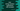# Dart string contains method explanation with example## Dart string contains method explanation with example:

In this program, we will learn how to use contains method of dart string with examples. contains method is used to check if a Pattern is in a string or not. We can use this method with a character, substring or regex pattern.

### Definition of contains:

This method is defined as below:

``bool contains(Pattern other, [int startIndex = 0]);``

Here, we are passing one Pattern to check in the string. startIndex is the starting index from where we need to check the pattern. This is an optional value. If we don’t provide this index, it takes 0 i.e. it starts from the start of the string.

The return value of this method is boolean. It returns true if it finds the pattern in the string. Else, it returns false.

### Example of contains:

Let’s try contains with an example.

``````int main(){
var givenStr = "Hello World";
print(givenStr.contains("World"));
print(givenStr.contains('W'));
print(givenStr.contains('2'));
print(givenStr.contains('H',1));
print(givenStr.contains(new RegExp(r'[A-Z]')));
}``````

It will print:

``````true
true
false
false
true``````

Here,

• 1st print statement prints true because givenStr contains the substring World
• 2nd print statement prints true because givenStr contains the character W
• 3rd print statement prints false because givenStr doesn’t contain the number 2
• 4th print statement prints false because H is not found after index 1
• 5th print statement prints true because we have character between A to Z, which is defined by the regular expression.